# QlikView Documents

Documents for QlikView related information.

Announcements
Talk to Experts Tuesday, Live Q&A, September 22: Moving from QlikView to Qlik Sense. REGISTER

# Set expression and dollar expansion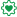Employee

## Set expression and dollar expansion

Quite often I see examples in the community where dollar expansions are used in set expressions. Nothing wrong with using variables to populate expressions, but it is easy to make mistakes if you do not consider what value the dollar expansion will actually expand into the expression. The example below gives a brief introduction to how you can validate that the dollar expansion is accurate and the value you are expecting.

In the example below I have loaded a calendar of 2014, and added a Max() aggregation that shows the highest day in each month.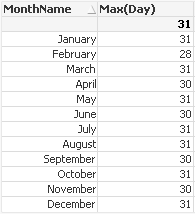Now I move the Max() aggregation into a set expression, where I have a set modifier that selects the max day.

count({<Day={"\$(=Max(Day))"}>} Day)

The result will be as below, where there is a 1 for every moth that has 31 days and a 0 for the other months. Notice that the expression label shows exactly which set expression has been evaluated, and that the set modifier is Day=31 and not the actual aggregation.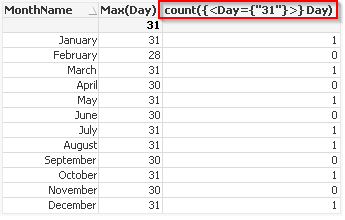This shows that the dollar expansion is done first and then the expression is evaluated in the chart. The aggregation in the set modifier will therefore only be evaluated once. The expanded value is also equal to the expression total of the expanded aggregation.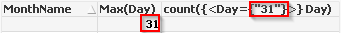Leave the expression label blank for troubleshooting or validation, so that you can see the fully expanded expression in the chart.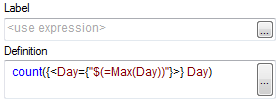For longer expression, or to avoid clearing the expression label, simply move the expanded aggregation into a separate expression and look at its Expression Total. This is set in the expression Total Mode settingLabels (5)

• ### QlikView ApplicationsContributor III

Hi @ToniKautto, a question:

why count({<Day={"\$(=Max(Day))"}>} Day) is not equal to write:

count({<Day={"=\$(=Max(Day))"}>} Day)?

It's seem to have another behavior..Partner

Set modifiers starting with = sign evaluate as booleans, so in this case they will select all values in Day for which the expression after = evaluates to True when aggregated by Day).

Well, in this case the expression is effectively this:
count({<Day={"=31"}>} Day)
which is true in every case, so it will just count all days.

Version history
Revision #:
1 of 1
Last update:
‎2015-05-05 08:34 PM
Updated by: